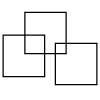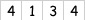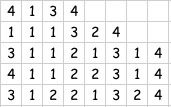#### You may also like### Three Squares

What is the greatest number of squares you can make by overlapping three squares?### Two Dice

Find all the numbers that can be made by adding the dots on two dice.### Biscuit Decorations

Andrew decorated 20 biscuits to take to a party. He lined them up and put icing on every second biscuit and different decorations on other biscuits. How many biscuits weren't decorated?

# Count the Digits

## Count the Digits

We can do all sorts of things with numbers - add, subtract, multiply, divide ...

Most of us start with counting when we are very little. We usually count things, objects, people etc. In this activity we are going to count the number of digits that are the same.

Rule 1 - The starting number has to have just three different digits chosen from $1, 2, 3, 4$.
Rule 2 - The starting number must have four digits - so thousands, hundreds, tens and ones.
For example, we could choose $2124$ or $1124$.

So when we've got our starting number we'll do some counting. Here is a worked example.

Starting NumberWe then count in order the number of $1$s, then the number of $2$s, then $3$s and lastly $4$s, and write it down as shown here.So the first count gave one $1$, one $3$ and two $4$s.
You may see that this has continued so the third line shows that the line above had three $1$s, one $2$, one $3$ and one $4$.
The fourth line counts the line above giving four $1$s, one $2$, two $3$s and one $4$.

And so it goes on until ... until when?
Your challenge is to start with other four digit numbers which satisfy the two rules and work on it in the way I did.
Tell us what you notice.

What happens if you have five digits in the starting number?

Got a solution? Then click on Submit a Solution above.

### Why do this problem?

This activity, in line with the theme for this month, offers an 'action' to perform on a group of numbers which pupils can continue and explore.  Or, you could think of the writing down of the 'description' of a sequence as an action performed on that sequence.  It might particularly appeal to those pupils who enjoy number work but who are perhaps not used to succeeding in this area.

### Possible approach

Introduce the task by taking an example and work it through with the group/class of pupils, emphasising how careful we have to be with the simple act of counting.

Give them plenty of time to explore their own choice of numbers before bringing them together to share findings. Depending on the age and experience of the learners, you may like to give them a separate sheet of paper simply to note down anything they notice as they work. Encourage them not to rub out as they go along so they have a record of their thoughts, to some extent.

A whole-group discussion could focus on what they notice and what other questions they might have as a result of working on this task. Some children might be keen to try to explain their findings. Do encourage them in this, even if you are not sure of the reasons yourself. Admitting your possible uncertainty will spur them on!

### Key questions

Tell me about what you see happening.
What will you do now?
Can you make any predictions before you start the next one?

### Possible extension

Change the rules so that only odd numbers are available, for example:
Rule 1 - The starting number must have just three different digits chosen from $1, 3, 5, 7$ Rule 2 - The starting number should have four digits, so thousands, hundreds, tens and ones.
For example, $3155$ or $1135$.
Some children might like to find out about 'Golomb sequences' which are related to this task.

### Possible support

Some pupils may need help in carefully counting the number of occurrences of each digit. It might, therefore, be useful for children to work in pairs so that someone else is always checking the counting.